• 偏微分方程组求解
千次阅读
2019-07-06 15:53:35

偏微分方程的数值解法，本质是偏微分方程的近似求解。虽然是近似，但整个近似过程满足严格的数学要求，如稳定性、收敛性、相容性等，并非随意的近似。由于时间，精力以及个人需求，仅秉着“理解不深究”的态度，进行了简单学习，并作如下笔记。因此下面难免有不严谨的地方，甚至错误，请大家适当取舍。（如有错误，请不吝赐教）

介绍的偏微分方程数值解法：有限差分法，有限元法（主要学习有限单元法），变分法与加权余量法

有限差分法

（1）基本思想

根据偏微分的定义，利用差商值近似求解微分方程的解。
（2）常微分方程的差分格式

最常见的有Euler差分格式和Runge-Kutta格式，简单介绍如下

• Euler差分，有向前差分，向后差分，中心差分等；从几何角度，利用‘三角性面积’代替“不规则形状的真实面积”
• Runge-Kutta差分，利用两点中间的几个位置点，求这些点斜率的平均值；从几何角度，利用‘平均斜率的矩形”代替“不规则的真实面积”
• 为提高差分精度，及一些特殊要求，还有其他差分格式，如Gear方法，等

（3）偏微分方程的差分格式

偏微分方程是分类的，有椭圆形、双曲线性、抛物线形偏微分方程。不同方程有不同的差分格式，整体而言，差分格式分为显式差分和隐式差分。显示差分指根据“上一层“”推测“下一层”，逐层递推；隐式差分则需要根据求解层 “上下层” ，进行求解。此外，边界条件的网格也是重要问题。

•

----------------------------------------------------------------手动分割线---------------------------------------------------------

扩展阅读

【转】  有限差分法求解偏微分方程  https://blog.csdn.net/baimafujinji/article/details/53053747

更多相关内容
• 非稳态的偏微分方程组是一个比较难解决的问题，也是在热质交换等方面的常常遇到的问题，因此需要一套程序来解决非稳态偏微分方程组的数值解。数值计算
• DuFort-Frankel格式求解椭圆-抛物型偏微分方程组，matlab程序，其中椭圆用积分公式，抛物用DuFort-Frankel格式，多多指教
• TMU_BME_2013 Topic: 如何使用 MATLAB 求 解常微分方程组 a.What ? 微分方程 指描述未知函数的导数与自变 量之间的关系的方程未知函数是一元函 数的微分方程称作 常微分方程 未知函数 是多元函数的微分方程称作 ...
• 该程序利用矢量化松弛算法来求解线性偏微分方程。 请提交您对改进程序的任何建议（我最终希望包含一个收敛常数以实现 SOR 方法）。 例子： %这是一个如何将程序用于网格空间中具有随机点电荷的平行板电容器的示例： ...matlab
• 然而，只有很少的微分方程可以解析求解，尤其对于偏微分方程，能解析求解的种类更是寥寥可数。更多的微分方程可以采用数值法进行求解，只要精度足够高，就可以满足科学和工程上的需求。数值求解微分方程的基本思路是...

1 引言

微分方程是描述一个系统的状态随时间和空间演化的最基本的数学工具之一，其在物理、经济、工程、社会等各方面都有及其重要的应用。然而，只有很少的微分方程可以解析求解，尤其对于偏微分方程，能解析求解的种类更是寥寥可数。更多的微分方程可以采用数值法进行求解，只要精度足够高，就可以满足科学和工程上的需求。

数值求解微分方程的基本思路是先把时间和空间离散化，然后将微分化为差分，建立递推关系，然后利用计算机强大的重复计算能力，快速得到任意格点处的值。Python的Numpy、Scipy工具包可以很好地实现此功能，Matplotlib工具包则可以将求解结果画为非常直观的图形。

接下来，我们先以常微分方程的数值求解为例，引入差分的思想，再将其推广到偏微分方程中。

2 常微分方程的差分求解

一般地，一阶常微分方程可以写为首先，将连续的变量离散化，连续的函数化为离散的序列，则上述微分方程可以化为差分方程【1】从而我们得到递推关系有了递推关系和初始条件以后，就可以利用Python的强大计算功能，得到任意的的值了，下面我们通过一个具体的例子来说明。

2.1 RC回路放电问题

对于一个回路，我们有其中，分别为电流，电阻，电量和电容，利用，并定义，我们得到一个含初始条件的一阶常微分方程这个方程当然可以解析求解，得到。另一方面按照差分法，可以得到递推关系下面我们用Python进行数值求解，并和解析结果进行比较。

import numpy as np

import matplotlib.pyplot as plt

rc = 2.0 #设置常数

dt = 0.5 #设置步长

n = 1000 #设置分割段数

t = 0.0 #设置初始时间

q = 1.0 #设置初始电量

#先定义三个空列表

qt=[] #用来盛放差分得到的q值

qt0=[] #用来盛放解析得到的q值

time = [] #用来盛放时间值

for i in range(n):

t = t + dt

q1 = q - q*dt/rc #qn+1的近似值

q = q - 0.5*(q1*dt/rc + q*dt/rc) #差分递推关系

q0 = np.exp(-t/rc) #解析关系

qt.append(q) #差分得到的q值列表

qt0.append(q0) #解析得到的q值列表

time.append(t) #时间列表

plt.plot(time,qt,'o',label='Euler-Modify') #差分得到的电量随时间的变化

plt.plot(time,qt0,'r-',label='Analytical') #解析得到的电量随时间的变化

plt.xlabel('time')

plt.ylabel('charge')

plt.xlim(0,20)

plt.ylim(-0.2,1.0)

plt.legend(loc='upper right')

plt.show()图1：用改进的欧拉法差分得到的结果和解析结果的比较

在图1中给出了差分法得到的结果与解析法得到结果的比较，发现两者符合得很好，这说明对于这个问题，改进的欧拉法已经可以给出足够精确的结果。需要注意的是，这个微分方程本身比较简单，可以解析求解，而对于复杂得多的微分方程，没法解析求解，但是上述数值求解差分方法仍然是适用的。

3 偏微分方程的差分求解

有了差分代替微分的思想，接下来我们将其推广到偏微分方程的求解中。以一般二阶抛物型偏微分方程为例，一般的可以写为仍然是将时间和空间离散化，将微分化为差分，即其中分别为空间步长和时间步长，分别标记空间指标和时间指标，则我们得到差分方程由此得到递推关系下面我们考察一个具体的例子，一维热传导方程的求解。

3.1 一维热传导方程的求解

一维热传导方程是一个典型的抛物型二阶偏微分方程。设表示在时间，空间处的温度，则根据傅里叶定律(单位时间内流经单位面积的热量和该处温度的负梯度成正比)，可以导出热传导方程【2】其中称为热扩散率，分别为热导率，比热和质量密度，都是由系统本身确定的常量。

为了具体，设，设边界条件为设步长为：，从而，所以递推关系为图2：递推关系示意图

图2直观地给出了差分法求解偏微分方程的过程。先把时空坐标都离散化，根据递推关系，由下一行的三个蓝点的值可以给出上一行的一个红点的值，由于边界条件和初始条件(即最下方和两边的绿线)已知，所以按这个递推关系可以得到网格中的所有值。下面我们用Python代码来实现。

import numpy as np

import matplotlib.pyplot as plt

h = 0.1#空间步长

N =30#空间步数

dt = 0.0001#时间步长

M = 10000#时间的步数

A = dt/(h**2) #lambda*tau/h^2

U = zeros([N+1,M+1])#建立二维空数组

Space = arange(0,(N+1)*h,h)#建立空间等差数列，从0到3，公差是h

#边界条件

for k in arange(0,M+1):

U[0,k] = 0.0

U[N,k] = 0.0

#初始条件

for i in arange(0,N):

U[i,0]=4*i*h*(3-i*h)

#递推关系

for k in arange(0,M):

for i in arange(1,N):

U[i,k+1]=A*U[i+1,k]+(1-2*A)*U[i,k]+A*U[i-1,k]

上述代码中，我们首先把位于0-3中的空间等分为30份，位于0-1的时间等分为10000份，然后通过设置初始条件、边界条件和递推关系并借助for循环就得到了1个30*10000的二维数组，里面放着每个离散的时空点的温度值。

为了直观地展现温度随时空的变化关系，接下来开始画图，首先画出不同时刻温度随空间坐标的变化

#不同时刻的温度随空间坐标的变化

plt.plot(Space,U[:,0], 'g-', label='t=0',linewidth=1.0)

plt.plot(Space,U[:,3000], 'b-', label='t=3/10',linewidth=1.0)

plt.plot(Space,U[:,6000], 'k-', label='t=6/10',linewidth=1.0)

plt.plot(Space,U[:,9000], 'r-', label='t=9/10',linewidth=1.0)

plt.plot(Space,U[:,10000], 'y-', label='t=1',linewidth=1.0)

plt.ylabel('u(x,t)', fontsize=20)

plt.xlabel('x', fontsize=20)

plt.xlim(0,3)

plt.ylim(-2,10)

plt.legend(loc='upper right')

plt.show()图3：不同时刻的温度随空间坐标的变化

从图3可以看到，温度关于呈现轴对称分布，这是由初始条件造成的。另外，对每一点的空间坐标，随着时间的推移，温度越来越低。

接下来，我们来画出温度等高线来描述温度随任意时空点的变化

#温度等高线随时空坐标的变化，温度越高，颜色越偏红

extent = [0,1,0,3]#时间和空间的取值范围

levels = arange(0,10,0.1)#温度等高线的变化范围0-10，变化间隔为0.1

plt.contourf(U,levels,origin='lower',extent=extent,cmap=plt.cm.jet)

plt.ylabel('x', fontsize=20)

plt.xlabel('t', fontsize=20)

plt.show()图4：温度随时空坐标的变化，温度越高，颜色越红

在图4中，利用颜色的深浅来标记温度，温度越高，颜色越红。从中同样可以看到，温度随空间的分布关于轴对称，而且随着时间的推移，温度越来越低。

4 总结

在本文中，我们利用Python数值求解了常微分方程和偏微分方程，基本思想是先将连续的坐标离散化，然后将微分化为差分，由差分方程得到递推关系，然后利用计算机强大的重复计算能力得到任意格点处的函数值。虽然上面只算了两个例子，但是这种方法完全可以推广到任意偏微分方程的求解中。

在量子色动力学(QCD)中，由于强相互作用具有渐进自由的特性，所以在低能情况下没办法像QED那样使用微扰论计算，这时就要采用格点QCD的方法计算。其基本思想也是将时空离散化，然后从第一性原理的路径积分出发去计算。由于时空被离散化了，相当于人为地引入了一个最小时空距离，在傅里叶变换到动量空间后相当于引入了一个最大的动量截断，所以计算结果不会出现紫外发散，从而可以算到很高的精度，在一些情况下，格点的计算结果甚至比实验更精确。所以，将连续参数离散化，把微分化为差分的思想，是极其重要的。

【1】不同的算法对于方程右边具体取什么形式并不一样，从而精度也不一样。例如，欧拉法右边取得是；改进的欧拉法右边取的是；二阶Runge-Kutta法右边取的是；四阶Runge-Kutta法右边取的是，其中。这些算法的差别在于计算精度不同，并不改变差分的本质思想。为了具体，我们这里采用改进的欧拉法。

【2】傅里叶定律告诉我们单位时间通过单位面积的热量和该处的温度负梯度成正比，即，其中是热流，即单位时间通过单位面积的热量，为热导率，为温度。能量守恒定律告诉我们单位时间流出某闭合曲面的热量等于其内部减少的能量，即，其中表示单位体积的热容，而分别为质量密度和单位质量的热容(比热)，联合散度定理，我们得到，再将傅里叶定律带入，就得到了热传导方程，其中称为热扩散率。在一维的情况下，热传导方程就退化到了正文中的形式，即展开全文• 对于偏微分方程求解，Matlab提供了两种工具。第一种是pdepe()函数，它的特点是通用性好，不受求解阶次的限制，不足之处是只支持命令行的格式；第二种是PDE工具箱，它的特点是提供了一个GUI界面，简洁易懂可视，...

从写完上一篇常微分方程的求解到现在已经很长时间了，这周也一直忙于报到的各种事宜，无暇坐下来写些东西，趁着这个周末，终于完成了这个姊妹篇。

对于偏微分方程的求解，Matlab提供了两种工具。第一种是pdepe()函数，它的特点是通用性好，不受求解阶次的限制，不足之处是只支持命令行的格式；第二种是PDE工具箱，它的特点是提供了一个GUI界面，简洁易懂可视，可以从枯燥的编程中解脱出来，不足之处是使用有限制，只能求解二阶的PDE，且不支持偏微分方程组的求解。

(1)、首先，我们来介绍pdepe()函数的使用。

pdepe()函数的调用格式为：

sol=pdepe(m,@pdefun,@pdeic,@pdebc,x,t)

其中，@pdefun是PDE方程的函数描述，它必须写成下面这种固定的格式：这样，偏微分方程可以编写下面的函数描述，其入口为

[c,f,s]=pdefun(x,t,u,ux)

其中，pdefun为函数名；m,x,t就是对应于标准格式中的相关参数。

@pdebc是PDE的边界条件描述函数，必须先化成下面的标准形式：于是边值条件可以编写下面的函数描述为

[pa,qa,pb,qb]=pdebc(x,t,u,du)

其中a表示上边界，b表示下边界。

@pdeic是PDE的初值条件，必须化成下面的形式：我们使用下面的简单函数来描述为

u0=pdeic(x)

sol是一个三维数组，sol(:,:,i)表示ui的解。

接下来，我们举一个例子来说明pdepe()函数的使用(由于没办法插入公式，截图如下)：经过上面的分析，我们使用如下程序求解：

function pde

%主函数

x=0:0.05:1;

t=0:0.05:2;

m=0;

sol=pdepe(m,@mpde,@mpic,@mpbc,x,t);

surf(x,t,sol(:,:,1))

figure;

surf(x,t,sol(:,:,2))

function

[c,f,s]=mpde(x,t,u,du) %给出偏微分方程的函数描述

c=[1;1];

f=[0.024*du(1);0.17*du(2)];

temp=u(1)-u(2);

s=[-1;1].*(exp(5.73*temp)-exp(-11.46*temp));

function

[pa,qa,pb,qb]=mpbc(xa,ua,xb,ub,t) %边界条件描述

pa=[0;ua(2)];

qa=[1;0];

pb=[ub(1)-1;0];

qb=[0;1];

function u0=mpic(x)

%初值描述

u0=[1;0];

结果如下：(2)、PDE工具箱的使用

在Matlab的Command Window中键入pdetool即可调出求解界面。界面求解比较简单，具体的使用方法这里就不做介绍了。

展开全文• 文章目录(1)偏微分方程的类型(二阶)(2)抛物线型1.显式法2.Crank-Nicholson隐式算法 (3)双曲线型(4)椭圆型 (1)偏微分方程的类型(二阶) a∂2u∂x2+b∂2u∂y∂x+c∂2u∂x2+d∂u∂x+e∂u∂y+fu+g=0a\frac{\partial^2u}{\...

# (1)偏微分方程的类型(二阶)

a ∂ 2 u ∂ x 2 + b ∂ 2 u ∂ y ∂ x + c ∂ 2 u ∂ x 2 + d ∂ u ∂ x + e ∂ u ∂ y + f u + g = 0 a\frac{\partial^2u}{\partial x^2}+b\frac{\partial^2u}{\partial y\partial x}+c\frac{\partial^2u}{\partial x^2}+d\frac{\partial u}{\partial x}+e\frac{\partial u}{\partial y}+fu+g=0

• b 2 − 4 a c < 0 b^2-4ac<0 椭圆
• b 2 − 4 a c = 0 b^2-4ac=0 抛物线
• b 2 − 4 a c > 0 b^2-4ac>0 双曲线

# (2)抛物线型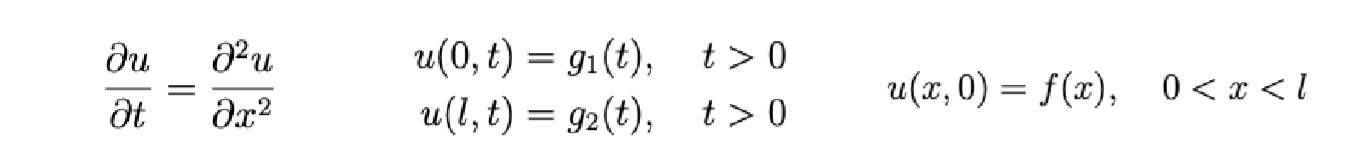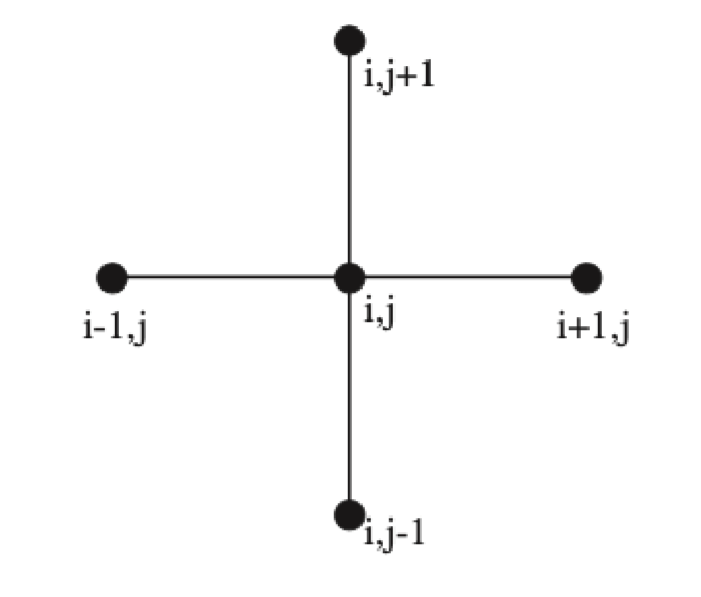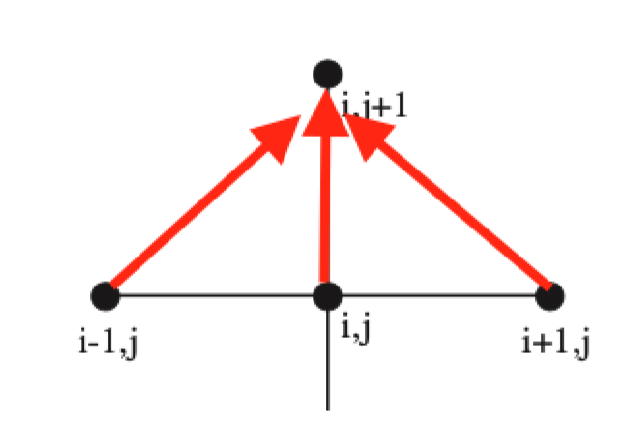## 1.显式法

• 求解思想：通过差分的方法一排一排向上推。
• 做划分并代入方程 u i , j + 1 − u i , j k = u i + 1 , j − 2 u i , j + u i − 1 , j h 2    ( Δ x = h , Δ t = k ) \frac{u_{i,j+1}-u_{i,j}}{k}=\frac{u_{i+1,j}-2u_{i,j}+u_{i-1,j}}{h^2}~~(\Delta x=h,\Delta t=k)
• 通过化简得到 u i , j + 1 = r u i − 1 , j + ( 1 − 2 r ) u i , j + r u i + 1 , j    ( r = k h 2 ) u_{i,j+1}=ru_{i-1,j}+(1-2r)u_{i,j}+ru_{i+1,j}~~(r=\frac{k}{h^2})
• 具体推的步骤大概如下：
• 由于已知 u ( x , 0 ) = f ( x ) u(x,0)=f(x) ，因此相当于知道 u 0 , 0 , u 1 , 0 , u 2 , 0 … u_{0,0},u_{1,0},u_{2,0}\dots
• 通过上面的公式就可以推出来 u 1 , 1 , u 2 , 1 , u 3 , 1 … u_{1,1},u_{2,1},u_{3,1}\dots ，注意由于已知左边界和右边界，因此 u 0 , 1 u_{0,1} 也知道，所以第二排就可以全部推出来。
• 通过上面的方式可以求出区域内全部的数值解。

## 2.Crank-Nicholson隐式算法

• 求解思想：也是一排一排向上推，但是这次是使用线性方程组一次性求出一排。
• 这里采用相同的划分方式，但是代入不同的差分方程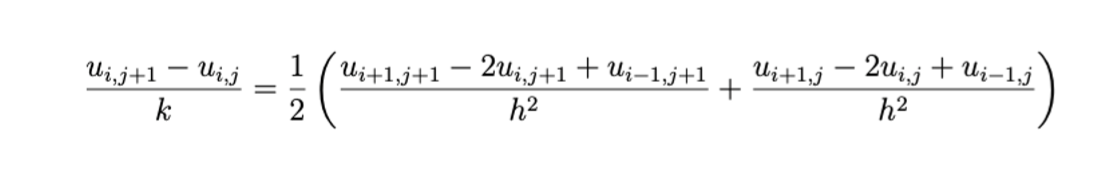• 通过化简得到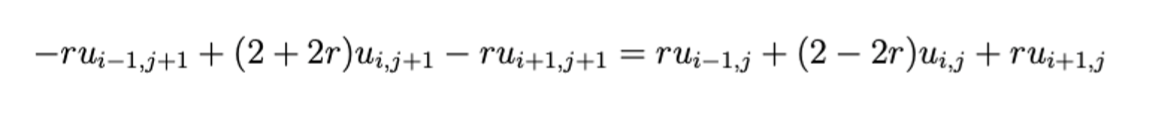• 具体推的步骤大概如下：
• 由于已知 u ( x , 0 ) = f ( x ) u(x,0)=f(x) ，因此相当于知道 u 0 , 0 , u 1 , 0 , u 2 , 0 … u_{0,0},u_{1,0},u_{2,0}\dots
• 通过上面的公式就可以推出来方程 − r u i − 1 , 1 + ( 2 + 2 r ) u i , 1 − r u i + 1 , 1 = c     ( c 是 一 个 常 数 ) -ru_{i-1,1}+(2+2r)u_{i,1}-ru_{i+1,1}=c~~~(c是一个常数) ，注意由于已知左边界和右边界，所以这个其实就转化成在一维上的差分问题，最后列出全部的方程构成方程组求解即可。
• 通过上面的方式可以求出区域内全部的数值解。
• 一个例子：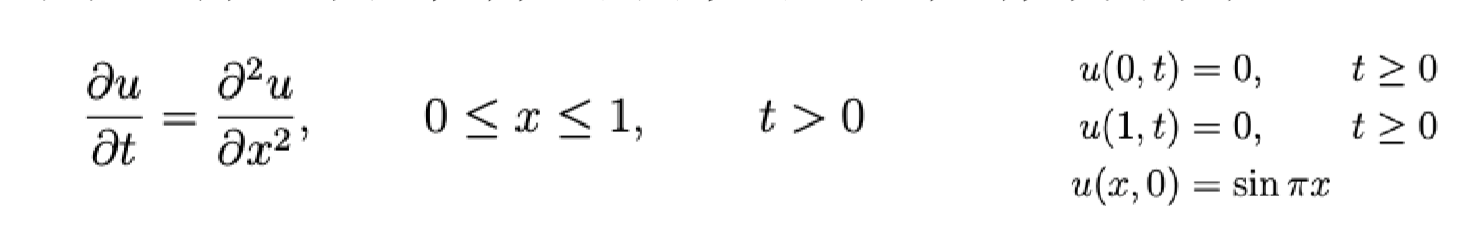• 做划分并且代入差分方程
k = 0.01 , h = 0.1 k=0.01,h=0.1
− u i − 1 , j + 1 + 4 u i , j + 1 − u i + 1 , j + 1 = u i − 1 , j + u i + 1 , j -u_{i-1,j+1}+4u_{i,j+1}-u_{i+1,j+1}=u_{i-1,j}+u_{i+1,j}
• 进行求解(这里利用了对称性，在 x = 0.5 x=0.5 两边是对称的，将 j = 0 j=0 隐去，并根据对称性将 u 6 u_6 替换成 u 4 u_4 )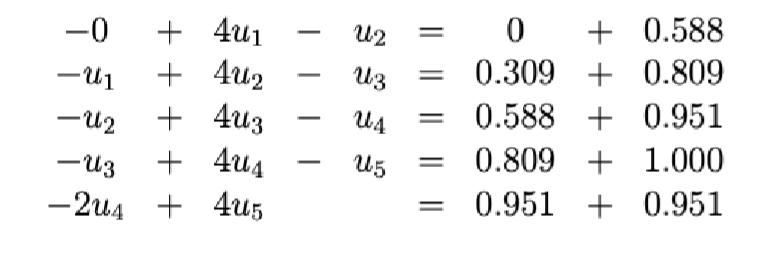# (3)双曲线型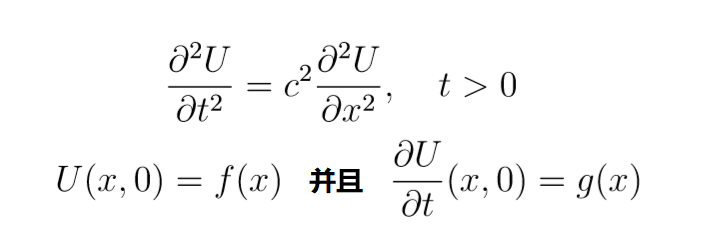• 得到的差分方程为 ( r = k h r=\frac{k}{h} 注意和之前的定义不同)：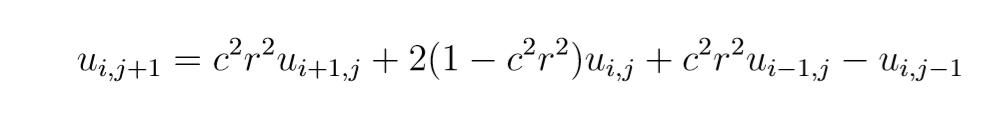• 划分需要满足一定的条件 k h ≤ 1 c \frac{k}{h}\le\frac{1}c{}
• 具体求解按照之前类似的方法即可。

# (4)椭圆型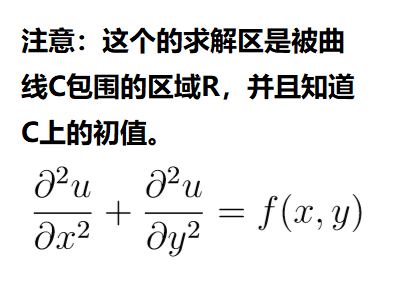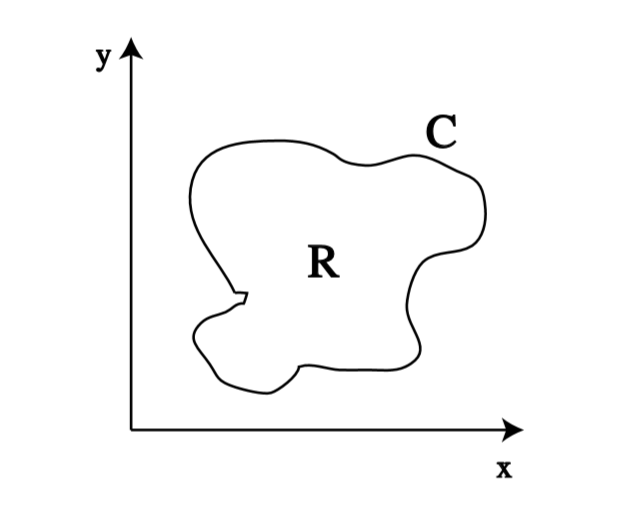• 得到的差分方程为 (这里取 k k h h 相等)：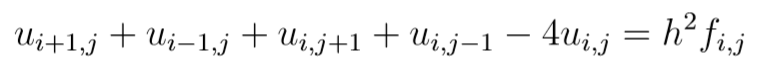• 求解
• 可以采用类似之前的隐式或者显式方法求解。
• 可以采用迭代法求解，比如雅克比迭代，转换成下面的迭代式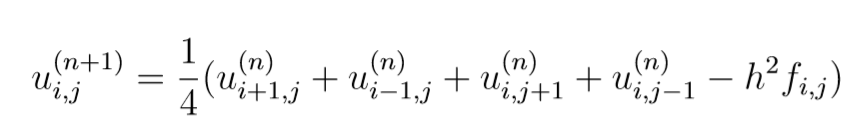展开全文机器学习
•matlab
• Solve[{a x + b y == 1, x - y == 2}, {x, y}]
• pde1dM在单个空间变量和时间内求解偏微分方程（PDE）系统。输入与MATLAB函数pdepe基本兼容。许多pdepe示例将与pde1dM配合使用，只需进行一些小的更改。 pde1dM solves systems of partial differential equations ...
• Matlab求解微分方程()及偏微分方程()
• 二阶椭圆偏微分方程实例求解(附matlab代码).docx 《微分方程数值解法》期中作业实验报告二阶椭圆偏微分方程第一边值问题姓名：学号：班级：2013年11月19日1二阶椭圆偏微分方程第一边值问题摘要对于解二阶椭圆偏微分...
• ## Python解偏微分方程

千次阅读 2021-07-14 16:39:57
泊松问题，包括偏微分方程 − ∇ 2 u = f -\nabla^{2} u=f −∇2u=f 和 ∂ Ω \partial \Omega ∂Ω 上的边界条件 u = u D u=u_{\mathrm{D}} u=uD​，是边界值问题的一个例子，在开始使用 FEniCS 解决它之前必须...python数值
• (1)PDEtool（GUI）求解偏微分方程的一般步骤 在Matlab命令窗口输入pdetool，回车，PDE工具箱的图形用户界面(GUI)系统就启动了.从定义一个偏微分方程问题到完成解偏微分方程的定解，整个过程大致可以分为六个阶段matlab
• 用python科学计算解偏微分方程，特别是用高斯迭代法计算拉普拉斯方程 用python科学计算解偏微分方程，特别是用高斯迭代法计算拉普拉斯方程python
• 写了个MATLAB的小程序，用特征线法求解 偏微分方程组。[XX,TT]=meshgrid(0:0.4:4,0:0.1:1);N=size(XX,2);T=size(XX,1);u1=zeros(T,N);u2=zeros(T,N);dx=0.4;dt=0.1;ds=0.1;u10=1:N;u20=(N:-1:1); % initial valueu...
• （最新）Matlab求解微分方程组及偏微分方程组
• Matlab求解微分方程组及偏微分方程组.doc
• 该模型是求解系数偏微分方程的好例子，神奇的大千世界都可在计算机上完美地重现！
• 由菲克第二定律可以得到动态扩散的偏微分方程求解可以得到浓度分布和流出曲线。不确定这个问题有没有解析解，不过数值求解是一种较为通用的解决方法。fipy是目前难得的还活着的PDE求解python包，作者根据官方示例...
• 不连续伽辽金法是一种求解各种偏微分方程的数值方法。 此代码的开发基于由Jan S. Hesthaven和Tim Warburton在其著作Nodal Discontinuous Galerkin Method 中开发的MATLAB代码版本。 此代码是使用 python 库开发的，...
• 偏微分方程组的MATLAB解法.pdf第第3737 卷第卷第23 期期··学术学术 湖南农机湖南农机 年年35 月月第37 卷第3 期 张向利：户县拖拉...
• 该方法可以快速有效地求解具有初始边界值（IBVP）的线性和非线性偏微分方程。 根据边界条件，将初始条件扩展为傅立叶级数。 之后，将IBVP转换为K域中的迭代关系。 可以得到级数解或精确解。 通过比较FDTM获得的结果...
• 赡芄酵频加写砦螅绻凑漳愕汲龅墓郊扑悖峒扑愠鲂橹担缓蟛煌５乃姥贰Ｈ缃玴u3/pt的最后k*Ac前面的负号改为正号则有解。从你的边界条件来看，似乎这个分量也应该跟其他两个应该是不一样的。...
• ## matlab偏微分方程工具箱求解

千次阅读 多人点赞 2020-12-14 18:13:50
偏微分方程组的matlab求解语句 ​ 该命令用以求解以下的PDEPDEPDE方程式： c(x,t,u,∂u∂x)∂u∂t=x−m∂(xmf(x,t,u,∂u∂x))∂x+s(x,t,u,∂u∂x) c(x,t,u,\frac{\partial u }{\partial x})\frac{\partial u}{\...matlab
• 用四阶龙格库塔法和自适应变步长法求解偏微分方程组
• 对于偏微分方程求解，Matlab提供了两种工具。第一种是pdepe()函数，它的特点是通用性好，不受求解阶次的限制，不足之处是只支持命令行的格式；第二种是PDE工具箱，它的特点是提供了一个GUI界面，简洁易懂可视，......